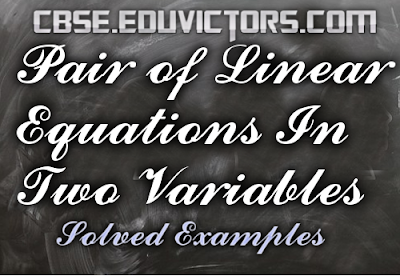## Wednesday, 8 August 2018

### CBSE Class 10 - Pair of Linear Equations In Two Variables - Solved Examples (#cbsenotes)(#eduvictors)

Pair of Linear Equations In Two Variables
Solved Examples

☛Following questions have been asked in previous years of CBSE board examination.

Q1: Solve the following system of linear equations by elimination method (equating the coefficients).
6 (ax + by) = 3a + 2b
6 (bx – ay) = 3b – 2a

Given equations :
6 (ax + by) = 3a + 2b ...(1)
6 (bx – ay) = 3b – 2a ...(2)

multiply eqn. (1) by a and equation (2) by b, and add, we get

6a²x + 6aby = 3a² + 2ab
6b²x – 6aby = 3b² – 2ab
────────────────────────
6 (a² + b²)x = 3(a + b)
────────────────────────

⇒ x = ½

Put x = ½ in equation (1), we have

6a × ½ + 6by = 3a + 2b

6by = 2b
y = ⅓

∴ x = ½ and y = ⅓        (Answer)

Q2: 2 tables and 3 chairs together cost Rs. 2000 whereas 3 tables and 2 chairs together cost ₹2500. Find the total cost of 1 table and 5 chairs.

Answer: Let the cost of a table be Rs. x and that of a chair be Rs. y.
According to given question,
2x + 3y = 2000 ...(1)
3x + 2y = 2500 ...(2)

Adding eqn. (1) and (2), we get
5x + 5y = 4500
x + y = 900 ...(3)

Subtracting eqn. (1) from eqn. (2), we get
x – y = 500 ...(4)

Adding eqn. (3) and eqn. (4), we get

2x = 1400
x = 700

Using x = 700 in eqn. (3), we get

700 + y = 900
y = 200
Cost of 1 table = ₹ 700 and cost of 1 chair = ₹ 200.

Hence, cost of 1 table and 5 chairs =  (x + 5y) = ₹ 700 + 5 (200) = ₹ 1700 (Answer)

Q3: Two angles are supplementary The larger angle is 3° less than twice the measure of the smaller angle. Find the measure of each angle.

Answer: Let the larger supplementary angle = x°
Smaller supplementary angle = y°

According to the question,
x = 2y – 3 ... (1)

Sum of the supplementary angles is 180°

x + y = 180 ... (2)

Subtract eqn. (1) from eqn. (2), we get

x + y = 180
x – 2y = -3
-   +
─────────────
3y = 183
─────────────

y = 61

Substitute the value of y in (1) or (2)
x + y = 180
x + 61 = 180
x = 119°

Two angles are 119° and 61°. (Answer)

Q4: 5 books and 7 pens together cost ₹79 whereas 7 books and 5 pens together cost ₹ 77. Find the total cost of 1 book and 2 pens.

Let the cost of a book be ₹ x and that of a pen be ₹ y. Then,
5x + 7y = 79 ..... (1)
and,
7x + 5y = 77 ....  (2)

Multiply equation (1) by 5 and equation (2) by 7, we get

25x + 35y = 395 ...... (3)
49x + 35y = 539 .......(4)

Subtracting equation (3) by equation (4), we get
49x - 25x = 539 - 395

24x = 144
x = 6
∴ Cost of a book = ₹ 6

Put x = 6 in equation (1), we get

5 × 6 + 7y = 79
30 + 7y = 79

7y = 79 - 30
7y = 49
y = 7

∴ Cost of a pen  ₹ 7

∴ Cost of 2 pens = 2 × 7 = ₹ 14

Hence, the total cost of 1 book and 2 pens = 6 + 14 = ₹ 20 (Answer)

CH 2: Polynomials (Study Points)
CH 2: Polynomials (NCERT Ex 2.1)
CH 2: Polynomials (NCERT Ex 2.2)

CH 3: Linear Equations In Two Variables (Revision Assignment)
CH 3: Linear Equations In Two Variables (Solved Examples)

CH 4 : Quadratic Equations (Ex 4.1)
CH 4 : Quadratic Equations (NCERT Ex 4.2)